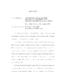## Asymptotic problems for stochastic processes and related differential equationsIt is well known that solutions of classical initial--boundary problems for second order parabolic equations depend continuously on the coefficients if the coefficients converge to their limits in a strong enough topology. In case of one spatial variable, we consider the question of the weakest possible topology providing convergence of the solutions. Convergence of solutions of PDE's is equivalent to weak convergence of corresponding diffusion processes. In general, continuous Markov processes corresponding to the generalized second order differential operators introduced by W. Feller can appear as limiting processes. In other words, the infinitesimal generator of limiting processes need not be a classical second order elliptic differential operator but instead can be a generalized in the sense of W. Feller \cite{WF1}. Following Freidlin and Wentzell's paper \cite{FW2}, where processes in open intervals were considered, we study the necessary and sufficient conditions for the weak convergence of one dimensional Markov processes in closed intervals. We provide conditions that guarantee the convergence of solutions of initial--boundary value problems for parabolic equations. Furthermore, necessary and sufficient conditions of weak convergence can be easily verified. In a number of articles, it was proved that the solution of reaction diffusion equations with a certain nonlinearity term is close for large $t$ to a running wave solution. However, in general, one cannot always give a simple formula for the asymptotic speed as was done in the Kolmogorov, Petrovskii and Piskunov (KPP) case in $R^1$. We apply our results to wave front propagation in narrow, of width $\varepsilon\ll 1$, domains. Especially, we consider the wave front propagation problem in a narrow periodic domain of width $\varepsilon \ll 1$ with the reaction term of KPP type. We focus on how a smooth or non--smooth periodic boundary of a narrow tube can affect the asymptotic speed of wave front propagation. In particular, under a non--smooth periodic boundary, the limit of solutions of initial--boundary problems is expected to satisfy a parabolic equation with a generalized second order operator, instead of a classical second order elliptic one.